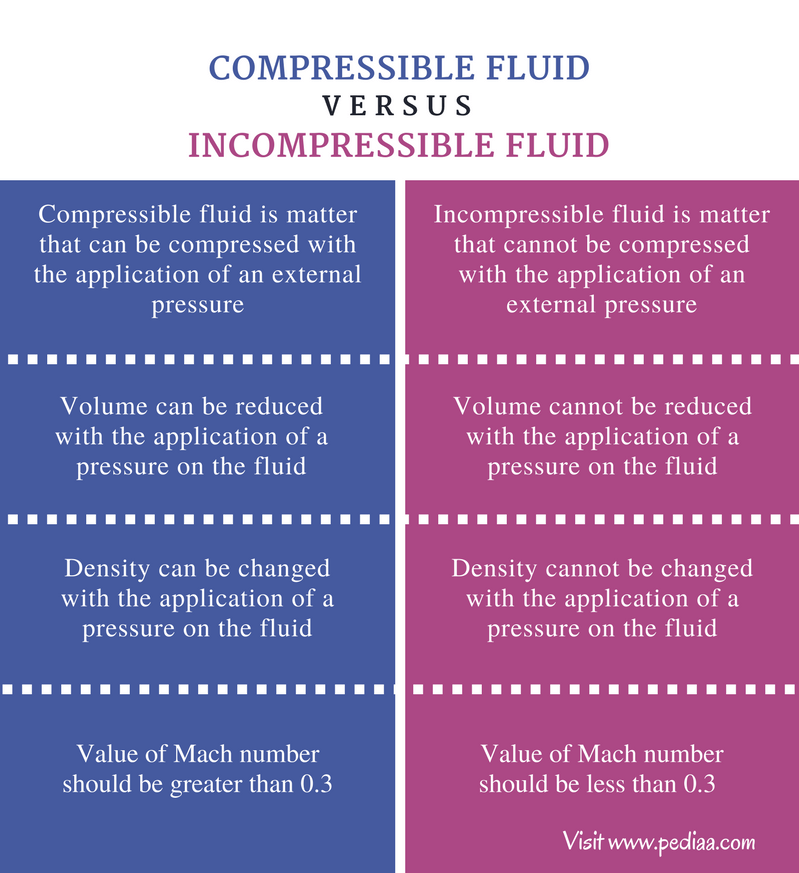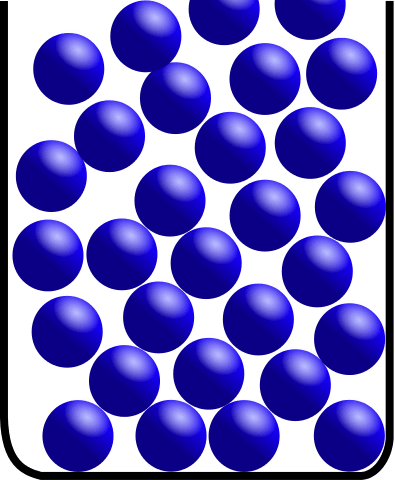# Difference Between Compressible and Incompressible Fluids

## Main Difference – Compressible vs Incompressible Fluids

Fluids are a subcategory of the matter which includes gases and liquids. Gases and liquids called fluids because of their ability to flow, ability to deform when a force is applied, and high fluidity. At the atomic level, fluids are composed of atoms or molecules which flow easily; they are not tightly packed and fluid obtains the shape of the container which it occupies. The main difference between compressible and incompressible fluid is that a force applied to a compressible fluid changes the density of a fluid whereas a force applied to an incompressible fluid does not change the density to a considerable degree. Although almost all fluids are compressible, liquids are known as incompressible fluids and gases are called compressible fluids.

### Key Areas Covered

1. What is Compressible Fluid
– Definition, Properties
2. What is Incompressible Fluid
Definition, Properties
3. What is the difference between Compressible and Incompressible Fluids
– Comparison of Key Differences

Key Terms: Fluid, Compressible Fluid, Incompressible Fluid, Liquid, Gas, Density, Mach Number## What is Compressible Fluid?

In general, gases (and plasma=ionized gas) are called compressible fluid. In normal temperature and pressure conditions, the volume or the density of a fluid does not change. But gases show variation in volume (hence in density) in the presence of even small variations in temperature or pressure. To name a particular fluid compressible, it should show a considerable change of density when a pressure or a force is applied.

In more advanced fluid dynamic terms, the ratio between the velocity of flow and the velocity of sound in the fluid is greater than 0.3 for compressible fluids. This ratio is also called Mach number.

At the molecular level, when a pressure is applied on a gas, the pressure affects the gas in all directions, causing the molecules of the gas to result in a high degree of collisions. These collisions give more time for the gas molecules to interact with each other and more attraction forces between molecules may occur. These attraction forces reduce the motion of gas molecules. This results in the compression of the gas.Figure 2: Gas Molecules in a Containe

## What is Incompressible Fluid?

Liquids are called incompressible fluid. The volume or the density of liquids is not changed easily when a pressure is applied on it. According to fluid dynamics, the ratio between flow velocity and the velocity of sound in the medium should be less than 0.3 for a fluid to be incompressible.  Hence, this ratio is less than 0.3 for liquids, making it an incompressible fluid.

Unlike in gases, the molecules or atoms of the liquids are more closely packed (not tightly packed as in solids). Thus, a pressure applied on liquid does not change the density to a considerable degree. In other words, the volume of the liquid is not reduced with an applied pressure on the liquid.

Although liquids are considered as incompressible according to the fluid dynamics, liquids are also compressible when a pressure is applied but the change of the density or volume is too small to be calculated. Hence, it is considered as an incompressible fluid.Figure: Liquid Molecules in a Container

## Differences Between Compressible and Incompressible Fluid

### Definition

Compressible Fluid: Compressible fluid is a matter that can be compressed with the application of an external pressure.

Incompressible Fluid: Incompressible fluid is a matter that cannot be compressed with the application of an external pressure.

### Volume

Compressible Fluid: The volume of compressible fluid can be reduced with the application of a pressure on the fluid.

Incompressible Fluid: The volume of incompressible fluid cannot be reduced with the application of a pressure on the fluid.

### Density

Compressible Fluid: The density of the compressible fluid can be changed with the application of a pressure on the fluid.

Incompressible Fluid: The density of the incompressible fluid cannot be changed with the application of a pressure on the fluid.

### Mach Number

Compressible Fluid: The value of Mach number should be greater than 0.3 for a compressible fluid.

Compressible Fluid: The value of Mach number should be less than 0.3 for an incompressible fluid.

### Conclusion

A fluid is a substance that can flow easily. A fluid has no definite shape and it takes the shape of the container which it is occupied. There are very weak attraction forces between molecules of the fluid. Gas and liquid phases are considered as fluids mainly due to their ability to flow. Gases are called compressible fluid whereas liquids are called incompressible fluid. The main difference between compressible and incompressible fluid is that a force applied to a compressible fluid changes the density of a fluid whereas a force applied to an incompressible fluid does not change the density to a considerable degree.

##### References:

1. Chang, Raymond, and Kenneth A. Goldsby. Chemistry. New York: McGraw-Hill, 2016. Print.
2. “Compressible fluid.” The Free Dictionary. Farlex, n.d. Web. Available here. 07 June 2017.
3. “What is a Fluid?” Boundless. Boundless, 08 Aug. 2016. Web. Available here. 07 June 2017.

##### Image Courtesy:

1. “Kinetic theory of gases” By I, Sharayanan (CC BY-SA 3.0) via Commons Wikimedia
2. “Liquid model” By Teilchenmodell Flüssigkeit.svg: Kaneiderdaniel.Original uploader was Kaneiderdaniel at de.wikipediaderivative work: Kayau (talk) – Teilchenmodell Flüssigkeit.svg (CC BY-SA 3.0) via Commons Wikimedia